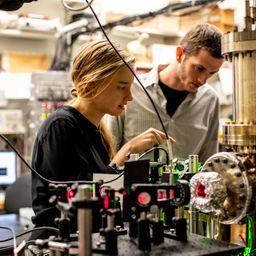## 4

Quantum Physics

Atomic Physics

### Discussion

You must be signed in to discuss.
##### Top Physics 103 Educators##### Christina K.

Rutgers, The State University of New Jersey##### Andy C.

University of Michigan - Ann Arbor

LB### Video Transcript

Okay, so for this problem, we know the first order. Max is a 12.6 degrees. So let's go ahead and write that down. And then the energy inter plainer spacing is 0.2 foreign. You know, meters, I'm gonna get that information down. So it's she's turned out any physics problem, which I'm sure, you know, by now you wanna get your givens down. Step one, we're all so you're just gonna look at the problem and probably possibly feel confused. So it's a nice practice to always do, and we want to know how many others could be observed in one angle. So they appear. So, uh, element. Well, let's find out. Let's explore that question. So in general, for us to see these interference, Max, is we need to de signed data equals I'm landed to be satisfied for some integer values of M, and we know what the 1st 1 is. And so, um, I guess what we could do is we could get lambda. Um and, uh, so we could get Lambda is to decide Data's Let's just go ahead and calculate that, and then then we can sort of, um yeah on then we can sort of figure everything out, Actually, do you even have to calculate Lambda? Um, you know, it's not too hard, and I think we're gonna have to calculate it, so I'll go. I didn't do that. So two times 20.2 for halftime. The minus nine times a sign of sex. Great. So we have 1.5 times for minus 10 meters a k 10 nanometers and basically for us to see Max, I mean, this has to be satisfied. And the big thing is that sign Can it goes? The magnitude goes from 0 to 1. So we basically want signed data to be from 0 to 1. So we want, um I'm lam da divided by to d to be by to be, ah, between zero and one and then And we know Landa formula for it is equal Thio It's just two d signed data, so these are gonna be data EMS. So Hey, let me rewind a little bit if I start to confuse you. Um, because I'm gonna use symbols and stuff because I really don't want to just do a bunch of calculations. What if I start to confuse you? Just know that you can just keep plugging in different numbers of letters for am their numbers for em. And make sure you got something lessons, your own one or else you can satisfy this a question. Um, but I'm gonna try to do it symbolically. So let's plug in for Lambda. We know Lambda is equal to Let's call this sign data one for the first order. So we know that, um, Lambda is equal to you know the number, but let's ride symbolically to de times the sign of beta one. Then we can also divide that by two D and then we need to multiply by. Um, so then these two teas nicely cancel. So it's just gonna be the sign data is going to be interred. Your multiples of signed data one. So, um, and then let's go. Actually, just calculate the sign of this angle, and so, um, the sign of 12.6 is 0.21 Great. So this is going like I like, um, so 0.21 So it's m times 0.21 It's not. We can see that, um, we need And then, like I was saying, kind of all the way down here. We need a sign to be less than one. So we need this to be less than one. So it's less than one for AM equals two, three, right? That's going to be 30.6 ish for is gonna be 0.8, but five. But to put it over one, so there should be a total of, uh, four peaks. I'll just go ahead and write it up here and box it up.University of Washington

#### Topics

Quantum Physics

Atomic Physics

##### Top Physics 103 Educators##### Christina K.

Rutgers, The State University of New Jersey##### Andy C.

University of Michigan - Ann Arbor

LB